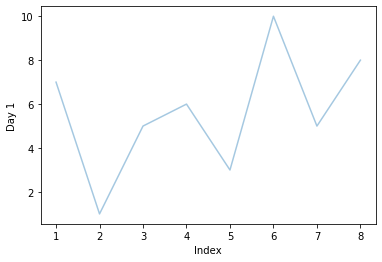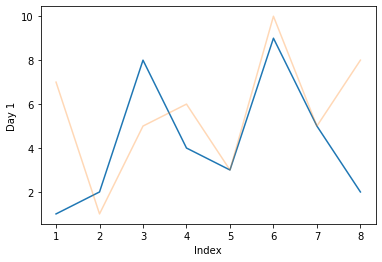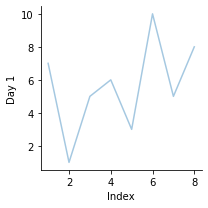# Seaborn 图的透明度

``````import pandas as pd
import matplotlib.pyplot as plt
import seaborn as sns
df = pd.DataFrame({"Day 1": [7,1,5,6,3,10,5,8],
"Index": [1,2,3,4,5,6,7,8],
})
sns.lineplot(data =df, y = 'Day 1', x= 'Index', alpha  = 0.4)
````````````import pandas as pd
import matplotlib.pyplot as plt
import seaborn as sns
df = pd.DataFrame({"Day 1": [7,1,5,6,3,10,5,8],
"Day 2" : [1,2,8,4,3,9,5,2],
"Index": [1,2,3,4,5,6,7,8]})
sns.lineplot(data =df, x = 'Index', y = 'Day 2', alpha  = 1)
sns.lineplot(data =df, x = 'Index', y = 'Day 1', alpha  = 0.3)
```````alpha` 参数也可用于不同的 FacetGrid、PairGrid 对象。对于此类对象，我们也可以使用 `map()` 函数中的参数。

``````import pandas as pd
import matplotlib.pyplot as plt
import seaborn as sns
df = pd.DataFrame({"Day 1": [7,1,5,6,3,10,5,8],
"Index": [1,2,3,4,5,6,7,8],
})
g = sns.FacetGrid(df)
g.map(sns.lineplot, 'Index', 'Day 1', alpha = 0.4)
``````